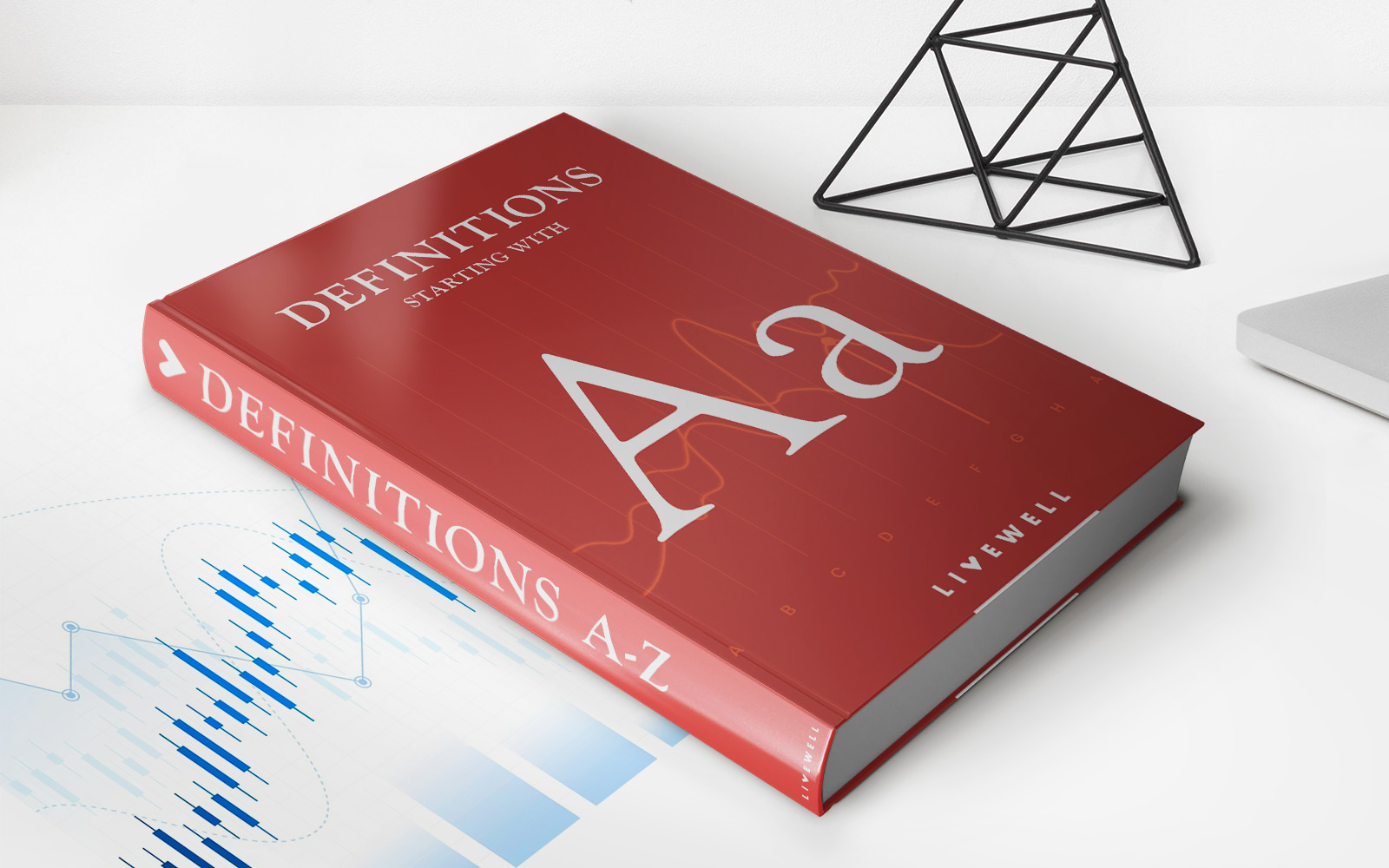Home>Finance>Absorbed Cost: Definition, Examples, ImportanceFinance

# Absorbed Cost: Definition, Examples, Importance

Learn about the definition, importance, and examples of absorbed cost in finance. Understand why absorbed cost is crucial for businesses' financial analysis and decision-making.

## Absorbed Cost: Definition, Examples, Importance

Have you ever wondered how businesses calculate the total cost of producing their goods or services? Well, that’s where the concept of absorbed cost comes into play. In this blog post, we will explore the definition of absorbed cost, provide examples of how it is calculated, and discuss its importance for businesses.

## Key Takeaways:

• Absorbed cost refers to the total cost of production, including both fixed and variable costs, that is allocated to each unit of output.
• It is calculated by dividing the total costs by the number of units produced, allowing businesses to determine the cost per unit of production.

### What is Absorbed Cost?

Absorbed cost is the term used to describe the total cost of manufacturing a product or providing a service. It encompasses both fixed costs, such as rent, utilities, and equipment depreciation, as well as variable costs, such as raw materials and direct labor expenses. By allocating these costs to each unit of output, businesses can accurately determine the cost per unit and make informed decisions about pricing and profitability.

### How is Absorbed Cost Calculated?

To calculate absorbed cost, businesses need to determine the total cost of production and the number of units produced. The calculation is relatively simple:

Absorbed Cost = Total Cost of Production / Number of Units Produced

This formula allows businesses to distribute the accumulated costs across all units of production, providing a more accurate representation of the expenses involved in making each unit.

### Examples of Absorbed Cost

Let’s take a look at a couple of examples to illustrate how absorbed cost works:

1. Jane’s Bakery: Jane owns a small bakery that produces 500 loaves of bread at a total cost of \$2,000. To calculate the absorbed cost, Jane divides the total cost by the number of units produced: \$2,000 / 500 = \$4. Therefore, the absorbed cost per loaf is \$4.
2. John’s Manufacturing Company: John’s company manufactures 1,000 widgets with a total cost of \$10,000. Applying the formula, John calculates the absorbed cost per widget as \$10,000 / 1,000 = \$10.

### Importance of Absorbed Cost

The concept of absorbed cost is crucial for businesses for several reasons:

1. Pricing: By understanding the absorbed cost per unit, businesses can set prices that cover their expenses while remaining competitive in the market.
2. Profitability Analysis: Absorbed cost allows businesses to assess the profitability of their products or services. By comparing the absorbed cost with the selling price, companies can determine their profit margin and make informed decisions about resource allocation.
3. Cost Control: Analyzing the absorbed cost helps businesses identify areas of inefficiency and control costs effectively. By monitoring the cost per unit, businesses can implement strategies to optimize production processes and reduce expenses.

Understanding absorbed cost is essential for businesses aiming to achieve financial success and make strategic decisions. By calculating and analyzing the absorbed cost accurately, businesses can achieve optimal pricing, evaluate profitability, and control costs efficiently.

• https://livewell.com/finance/absorbed-cost-definition-examples-importance/